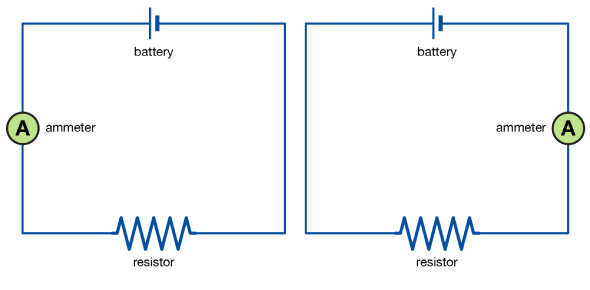# A Trivia Quiz On Electrical Circuits!

8 Questions | Total Attempts: 8351SettingsDo you think you know everything there is to know about electrical circuits? Do you think you can pass this quiz? An electrical circuit is an interconnectedness of electrical components. An electrical circuit is comprised of batteries, resistors, inductors, capacitors, switches, and transistors. An electrical system consists of a closed-loop, which is an entire route that an electric current can flow around. This quiz will help you better understand electrical circuits. Don't neglect to procure your certificate when you have completed the quiz.

• 1.
Which of the following describes a series circuit?
• A.

Current flows along each branch

• B.

Bulbs are all in a loop

• C.

If one bulb breaks the other one still works

• 2.
Which of the following describes a parallel circuit?
• A.

All bulbs light up together

• B.

If one bulb breaks the circuit is broken

• C.

Current flows along each branch

• 3.
What units does the electrical current have?
• A.

Amps

• B.

Volts

• C.

Power

• 4.
What is the symbol of a bulb?
• A.

A rectangle

• B.

A circle with an x

• C.

One big and one small line

• 5.
What type of circuit do we find in our houses?
• A.

Parallel

• B.

Series

• 6.
When you move your brain uses electrical signals. these are called
• A.

Nerve signals

• B.

Electrical signals

• C.

Impulses

• 7.
If your heart stops, doctors can start it again by using a
• A.

Electric shocker

• B.

Defibrillator

• C.

Pacemaker

• 8.
When you skin is wet, electricity can pass through you
• A.

Easier

• B.

Harder

• C.

The same

Related TopicsBack to top